International Tables for Crystallography (2006). Vol. D, ch. 3.3, pp. 393-448https://doi.org/10.1107/97809553602060000644

## Contents

• 3.3. Twinning of crystals  (pp. 393-448)
• 3.3.1. Crystal aggregates and intergrowths  (pp. 393-394) | html | pdf |
• 3.3.2. Basic concepts and definitions of twinning  (pp. 394-398) | html | pdf |
• 3.3.2.1. Definition of a twin  (p. 394) | html | pdf |
• 3.3.2.2. Essential addenda to the definition  (pp. 394-395) | html | pdf |
• 3.3.2.3. Specifications and extensions of the orientation relations  (pp. 395-397) | html | pdf |
• 3.3.2.3.1. Binary twin operations (twin elements)  (pp. 395-396) | html | pdf |
• 3.3.2.3.2. Pseudo n -fold twin rotations (twin axes) with(pp. 396-397) | html | pdf |
• 3.3.2.4. Notes on the definition of twinning  (pp. 397-398) | html | pdf |
• 3.3.3. Morphological classification, simple and multiple twinning  (pp. 398-399) | html | pdf |
• 3.3.3.1. Morphological classification  (pp. 398-399) | html | pdf |
• 3.3.4. Composite symmetry and the twin law  (pp. 399-402) | html | pdf |
• 3.3.4.1. Composite symmetry  (pp. 399-400) | html | pdf |
• 3.3.4.2. Equivalent twin laws  (pp. 400-401) | html | pdf |
• 3.3.4.3. Classification of composite symmetries  (p. 401) | html | pdf |
• 3.3.4.4. Categories of composite symmetries  (pp. 401-402) | html | pdf |
• 3.3.5. Description of the twin law by black–white symmetry  (pp. 402-403) | html | pdf |
• 3.3.6. Examples of twinned crystals   (pp. 403-412) | html | pdf |
• 3.3.6.1. Inversion twins in orthorhombic crystals  (p. 403) | html | pdf |
• 3.3.6.2. Twinning of gypsum  (pp. 403-404) | html | pdf |
• 3.3.6.3. Twinning of low-temperature quartz (α-quartz)  (pp. 404-405) | html | pdf |
• 3.3.6.3.1. Dauphiné twins  (p. 404) | html | pdf |
• 3.3.6.3.2. Brazil twins  (pp. 404-405) | html | pdf |
• 3.3.6.3.3. Combined Dauphiné–Brazil (Leydolt, Liebisch) twins  (p. 405) | html | pdf |
• 3.3.6.3.4. Japanese twins (or La Gardette twins)  (p. 405) | html | pdf |
• 3.3.6.4. Twinning of high-temperature quartz (β-quartz)  (pp. 405-406) | html | pdf |
• 3.3.6.5. Twinning of rhombohedral crystals  (p. 406) | html | pdf |
• 3.3.6.6. Spinel twins  (pp. 407-408) | html | pdf |
• 3.3.6.7. Growth and transformation twins of K 2 SO 4   (p. 408) | html | pdf |
• 3.3.6.8. Pentagonal–decagonal twins  (p. 408) | html | pdf |
• 3.3.6.9. Multiple twins of rutile  (pp. 408-409) | html | pdf |
• 3.3.6.10. Variety of twinning in gibbsite, Al(OH) 3   (pp. 409-410) | html | pdf |
• 3.3.6.11. Plagioclase twins  (p. 410) | html | pdf |
• 3.3.6.12. Staurolite  (pp. 410-411) | html | pdf |
• 3.3.6.13. BaTiO 3 transformation twins  (pp. 411-412) | html | pdf |
• 3.3.6.14. Twins of twins  (p. 412) | html | pdf |
• 3.3.7. Genetic classification of twins  (pp. 412-416) | html | pdf |
• 3.3.7.1. Growth twinning  (pp. 412-414) | html | pdf |
• 3.3.7.1.1. Twinning by nucleation  (pp. 412-413) | html | pdf |
• 3.3.7.1.2. Twinning during crystal growth  (pp. 413-414) | html | pdf |
• 3.3.7.2. Transformation twinning  (pp. 414-415) | html | pdf |
• 3.3.7.3. Mechanical twinning  (pp. 415-416) | html | pdf |
• 3.3.8. Lattice aspects of twinning  (pp. 416-422) | html | pdf |
• 3.3.8.1. Basic concepts of Friedel's lattice theory  (p. 417) | html | pdf |
• 3.3.8.2. Lattice coincidences, twin lattice, twin lattice index  (pp. 417-418) | html | pdf |
• 3.3.8.3. Twins with three-dimensional twin lattices (triperiodic' twins)  (pp. 418-419) | html | pdf |
• 3.3.8.4. Approximate (pseudo-)coincidences of two or more lattices  (pp. 419-420) | html | pdf |
• 3.3.8.5. Twin obliquity and lattice pseudosymmetry  (pp. 420-421) | html | pdf |
• 3.3.8.6. Twinning of isostructural crystals  (p. 422) | html | pdf |
• 3.3.8.7. Conclusions  (p. 422) | html | pdf |
• 3.3.9. Twinning by merohedry and pseudo-merohedry  (pp. 422-425) | html | pdf |
• 3.3.9.1. Definitions of merohedry  (pp. 422-423) | html | pdf |
• 3.3.9.2. Types of twins by merohedry and pseudo-merohedry  (pp. 423-425) | html | pdf |
• 3.3.9.2.1. Merohedral twins of lattice index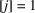(p. 423) | html | pdf |
• 3.3.9.2.2. Pseudo-merohedral twins of lattice index(p. 423) | html | pdf |
• 3.3.9.2.3. Twinning with partial lattice coincidence (lattice index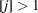)  (pp. 423-424) | html | pdf |
• 3.3.9.2.4. Twinning with partial lattice pseudo-coincidence (lattice index)  (pp. 424-425) | html | pdf |
• 3.3.9.3. Pseudo-merohedry and ferroelasticity  (p. 425) | html | pdf |
• 3.3.10. Twin boundaries  (pp. 426-444) | html | pdf |
• 3.3.10.1. Contact relations in twinning  (p. 426) | html | pdf |
• 3.3.10.2. Strain compatibility of interfaces  (pp. 426-430) | html | pdf |
• 3.3.10.2.1. Sapriel approach to permissible (compatible) boundaries in ferroelastic (non-merohedral) transformation twins  (pp. 427-428) | html | pdf |
• 3.3.10.2.2. Extension to non-merohedral growth and mechanical twins  (pp. 428-429) | html | pdf |
• 3.3.10.2.3. Permissible boundaries in merohedral twins (lattice index)  (pp. 429-430) | html | pdf |
• 3.3.10.2.4. Permissible twin boundaries in twins with lattice index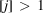(p. 430) | html | pdf |
• 3.3.10.3. Electrical constraints of twin interfaces  (pp. 430-432) | html | pdf |
• 3.3.10.3.1. Merohedral twins  (pp. 430-431) | html | pdf |
• 3.3.10.3.2. Non-merohedral twins  (p. 431) | html | pdf |
• 3.3.10.3.3. Non-pyroelectric acentric crystals  (pp. 431-432) | html | pdf |
• 3.3.10.4. Displacement and fault vectors of twin boundaries  (pp. 432-434) | html | pdf |
• 3.3.10.4.1. Twin displacement vector(pp. 432-433) | html | pdf |
• 3.3.10.4.2. Fault vectors of twin boundaries in merohedral twins  (pp. 433-434) | html | pdf |
• 3.3.10.4.3. Examples of fault-vector determinations  (p. 434) | html | pdf |
• 3.3.10.5. Examples of structural models of twin boundaries  (pp. 434-437) | html | pdf |
• 3.3.10.5.1. Aragonite, CaCO 3   (pp. 434-435) | html | pdf |
• 3.3.10.5.2. Dauphiné twins of-quartz  (pp. 435-436) | html | pdf |
• 3.3.10.5.3. Potassium lithium sulfate, KLiSO 4   (p. 436) | html | pdf |
• 3.3.10.5.4. Twin models of molecular crystals  (pp. 436-437) | html | pdf |
• 3.3.10.6. Observations of twin boundaries by transmission electron microscopy  (pp. 437-439) | html | pdf |
• 3.3.10.6.1. Anatase, TiO 2 (Penn & Banfield, 1998, 1999)  (p. 437) | html | pdf |
• 3.3.10.6.2. SnO 2 (rutile structure)  (p. 437) | html | pdf |
• 3.3.10.6.3.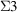(111) twin interface in BaTiO 3 [ cf. Section 3.3.8.3(iii)]  (p. 437) | html | pdf |
• 3.3.10.6.4.bicrystal boundaries in Cu and Ag  (pp. 437-438) | html | pdf |
• 3.3.10.6.5. Fivefold cyclic twins in nanocrystalline materials  (pp. 438-439) | html | pdf |
• 3.3.10.7. Twin textures  (pp. 439-442) | html | pdf |
• 3.3.10.7.1. Merohedral (non-ferroelastic) twins (see Sections 3.3.9 and 3.3.10.2.3)  (p. 439) | html | pdf |
• 3.3.10.7.2. Non-merohedral (ferroelastic) twins  (p. 439) | html | pdf |
• 3.3.10.7.3. Fitting problems of ferroelastic twins  (pp. 440-441) | html | pdf |
• 3.3.10.7.4. Tweed microstructures  (pp. 441-442) | html | pdf |
• 3.3.10.7.5. Twin textures in polycrystalline aggregates  (p. 442) | html | pdf |
• 3.3.10.8. Twinning dislocations  (pp. 442-443) | html | pdf |
• 3.3.10.9. Coherent and incoherent twin interfaces  (pp. 443-444) | html | pdf |
• 3.3.11. Glossary  (pp. 444-445) | html | pdf |
• References | html | pdf |
• Figures
• Fig. 3.3.1.1. Parallel intergrowth ( a ) of spinel octahedra and ( b ) of hexagonal quartz prisms  (p. 393) | html | pdf |
• Fig. 3.3.1.2. ( a ) Optical anomaly of a cubic mixed (K,NH 4 )-alum crystal grown from aqueous solution, as revealed by polarized light between crossed polarizers  (p. 393) | html | pdf |
• Fig. 3.3.2.1. Schematic illustration of the orientation relations of triclinic twin partners, see Section 3.3.2.3.1  (p. 395) | html | pdf |
• Fig. 3.3.2.2. As Fig. 3.3.2.1, ( a ) for twin element (iii) rational twofold twin axis' and ( b ) for twin element (iv) irrational twin mirror plane' (see text); common lattice row [ uvw ] for both cases  (p. 395) | html | pdf |
• Fig. 3.3.2.3. Illustration of the Kantennormalengesetz (complex twin) for twin elements (v) and (vi) (see text); common lattice row [ uvw ] for both cases  (p. 396) | html | pdf |
• Fig. 3.3.2.4. ( a ) Triple growth twin of orthorhombic aragonite, CaCO 3 , with pseudo-threefold twin axis  (p. 397) | html | pdf |
• Fig. 3.3.3.1. Schematic illustration of simple (polysynthetic) and multiple (cyclic) twins  (p. 398) | html | pdf |
• Fig. 3.3.4.1. Gypsum dovetail twin: schematic illustration of the coset of alternative twin operations  (p. 399) | html | pdf |
• Fig. 3.3.4.2. Twinning of an orthorhombic crystal with equivalent twin mirror planes (110) and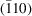(p. 400) | html | pdf |
• Fig. 3.3.6.1. Dovetail twin ( a ) and Montmartre twin ( b ) of gypsum  (p. 403) | html | pdf |
• Fig. 3.3.6.2. Distinction of the four different domain states generated by the three merohedral twin laws of low-quartz and of quartz homeotypes  (p. 405) | html | pdf |
• Fig. 3.3.6.3. The four variants of Japanese twins of quartz  (p. 406) | html | pdf |
• Fig. 3.3.6.4. Twin intergrowth of obverse' and `reverse' rhombohedra of rhombohedral FeBO 3   (p. 406) | html | pdf |
• Fig. 3.3.6.5. Contact growth twin of calcite with the same twin law as FeBO 3 in Fig. 3.3.6.4  (p. 407) | html | pdf |
• Fig. 3.3.6.6. Spinel (111) twins of cubic crystals (two orientation states)  (p. 407) | html | pdf |
• Fig. 3.3.6.7. Pseudo-hexagonal growth twin of K 2 SO 4 showing six sector domains in three orientation states  (p. 408) | html | pdf |
• Fig. 3.3.6.8. Pentagonal–decagonal twins  (p. 408) | html | pdf |
• Fig. 3.3.6.9. Various forms of rutile (TiO 2 ) twins, with one or several equivalent twin reflection planes {011}  (p. 409) | html | pdf |
• Fig. 3.3.6.10. Sixfold reflection twin of gibbsite, Al(OH) 3 , with equivalent (110) and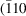), both as twin mirror and composition planes  (p. 410) | html | pdf |
• Fig. 3.3.6.11. Polysynthetic albite twin aggregate of triclinic feldspar, twin reflection and composition plane (010)  (p. 410) | html | pdf |
• Fig. 3.3.6.12. Pericline twin of triclinic feldspar  (p. 411) | html | pdf |
• Fig. 3.3.6.13. Twinning of staurolite  (p. 411) | html | pdf |
• Fig. 3.3.7.1. Orthoclase (monoclinic K-feldspar)  (p. 413) | html | pdf |
• Fig. 3.3.7.2. Photographs of (001) plates (20 mm diameter,1 mm thick) of NH 4 LiSO 4 between crossed polarizers  (p. 413) | html | pdf |
• Fig. 3.3.7.3. Mechanical twins of calcite, CaCO 3   (p. 415) | html | pdf |
• Fig. 3.3.8.1. Lattice relations oftwins of tetragonal crystals with primitive lattice  (p. 419) | html | pdf |
• Fig. 3.3.8.2. ( a ) A (110) silicon slice (10 cm diameter, 0.3 mm thick), cut from a Czochralski-grown tricrystal for solar-cell applications  (p. 419) | html | pdf |
• Fig. 3.3.10.1. ( a ) Classical description of mechanical twinning by homogeneous shear deformation  (p. 427) | html | pdf |
• Fig. 3.3.10.2. Boundaries B–B between 180° domains (merohedral twins) of pyroelectric crystals  (p. 431) | html | pdf |
• Fig. 3.3.10.3. Charged and uncharged boundaries B–B of non-merohedral twins of pseudo-hexagonal NH 4 LiSO 4   (p. 432) | html | pdf |
• Fig. 3.3.10.4. Lattice representation of twin displacement vectors  (p. 432) | html | pdf |
• Fig. 3.3.10.5. HRTEM micrograph of anatase, TiO 2 , with a (112) reflection twin boundary (arrows), viewed edge-on along(p. 433) | html | pdf |
• Fig. 3.3.10.6. Structural model of the (110) twin boundary of aragonite (after Bragg, 1924), projected along the pseudo-hexagonal c axis  (p. 435) | html | pdf |
• Fig. 3.3.10.7. Simplified structural model of a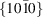Dauphiné twin boundary in quartz  (p. 435) | html | pdf |
• Fig. 3.3.10.8. KLiSO 4 : Bulk tetrahedral framework structures and models of (0001) twin boundary structures of phases III and IV  (p. 436) | html | pdf |
• Fig. 3.3.10.9. ( a ) HRTEM micrograph of a coherent (111) twin boundary in BaTiO 3 , projected along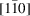(p. 438) | html | pdf |
• Fig. 3.3.10.10. ( a ) Schematic block diagram of abicrystal (spinel twin) for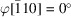(p. 438) | html | pdf |
• Fig. 3.3.10.11. HRTEM micrograph of a fivefold-twinned Ge nanocrystal (right) in an amorphous Ge film formed by vapour deposition on an NaCl cleavage plane  (p. 439) | html | pdf |
• Fig. 3.3.10.12. Illustration of space-filling problems of domains for a (ferroelastic) orthorhombicmonoclinic phase transition with an angle(exaggerated) of spontaneous shear  (p. 440) | html | pdf |
• Fig. 3.3.10.13. Thin section of tetragonal leucite, K(AlSi 2 O 6 ), between crossed polarizers  (p. 441) | html | pdf |
• Fig. 3.3.10.14. Twin textures generated by the two different hexagonal-to-orthorhombic phase transitions of KLiSO 4   (p. 441) | html | pdf |
• Fig. 3.3.10.15. Definition of the Burgers vector b of a twinning dislocation TD  (p. 443) | html | pdf |
• Tables
• Table 3.3.4.1. Gypsum, dovetail twins: coset of alternative twin operations (twin law), given in orthorhombic axes of the composite symmetry(p. 400) | html | pdf |
• Table 3.3.4.2. Reduced composite symmetries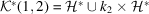and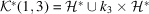for the orthorhombic example in Fig. 3.3.4.2  (p. 401) | html | pdf |
• Table 3.3.6.1. Orthorhombic inversion twins: coset of alternative twin operations (twin law)  (p. 403) | html | pdf |
• Table 3.3.6.2. Gypsum: cosets of alternative twin operations of the dovetail and the Montmartre twins, referred to their specific orthorhombic axes (subscripts D and M)  (p. 404) | html | pdf |
• Table 3.3.6.3. Dauphiné twins of α-quartz: coset of alternative twin operations (twin law)  (p. 404) | html | pdf |
• Table 3.3.6.4. The four different variants of Japanese twins according to Frondel (1962)  (p. 405) | html | pdf |
• Table 3.3.6.5. Plagioclase: albite and pericline twins  (p. 410) | html | pdf |
• Table 3.3.8.1. Lattice planes ( hkl ) and lattice rows [ uvw ] that are mutually perpendicular (after Koch, 2004)  (p. 418) | html | pdf |
• Table 3.3.8.2. Examples of calculated obliquitiesand lattice indices [ j ] for selected ( hkl )[ uvw ] combinations and their relation to twinning  (p. 421) | html | pdf |
• Table 3.3.9.1. Staurolite, 60° and 90° twins  (p. 425) | html | pdf |
• Table 3.3.10.1. Examples of permissible twin boundaries for higher-order merohedral twins ([j]> 1)  (p. 430) | html | pdf |